# Pareto Chart in Tableau

Pareto chart is basically based on the theory of “80-20” phenomena, where it means that 80% of the output is being generated by the 20% of the input. In terms of retail data, we can also say like this that 80% of revenue is from 20% of customers. Pareto chart is a combination of both bar and line graph in which bars are arranged in the descending order and line in the ascending cumulative total. Learn Tableau for Data Science.

Summary:
In this blog, I am going to create a Pareto chart in tableau using Sample-Superstore data.
To create a Pareto chart, we first need to know the dimension and measure. So, in this case, I am taking “Sub-Category” as dimension and “Profit” as a measure.

Following are the steps to create a Pareto chart in Tableau:

1. Connect to Sample-Superstore dataset: Open the Tableau Desktop and select the “Sample-Superstore” dataset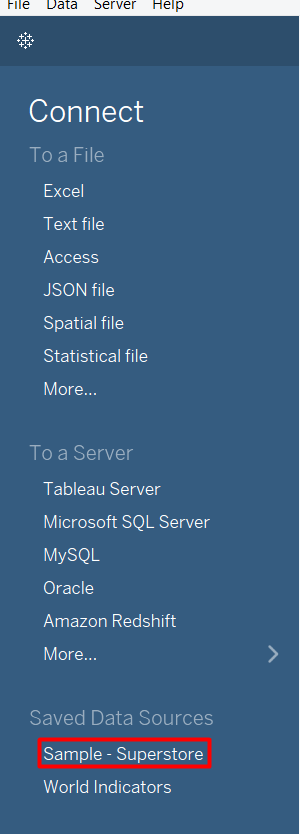2. Go to Sheet1: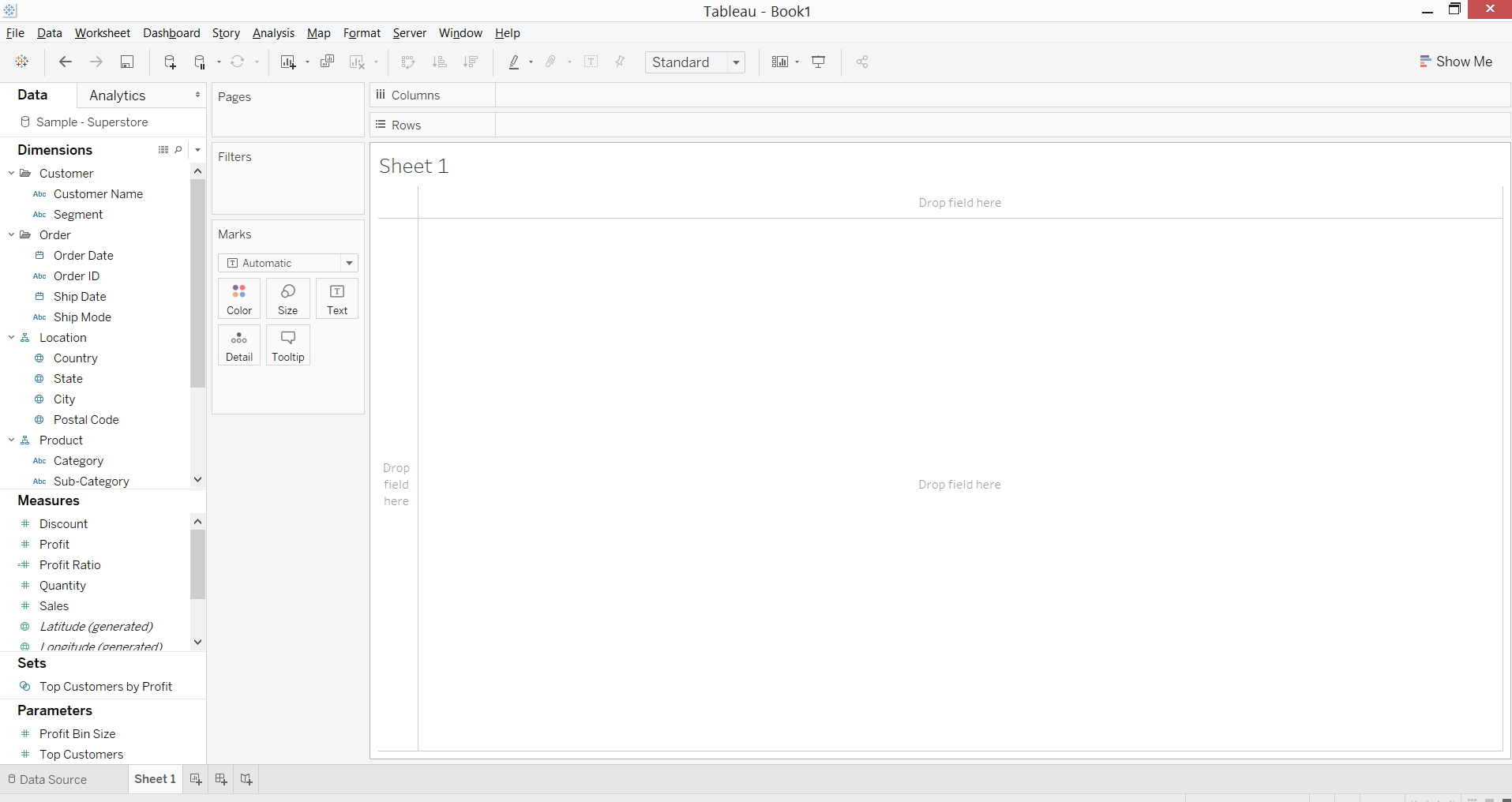3. Drag the “Sub-Category” dimension to “Columns” shelf and “Profit” measure to rows shelf4. Sort the “Sub-Category” in descending order based on “Profit” values5. Now, again drag the “Profit” measure to “Rows” shelf and put it on the right side after 1st “Profit” field
Also, make the chart as “Entire View”.6. Select the “Sum(Profit) (2)” in marks card and change the type of chart to “Line”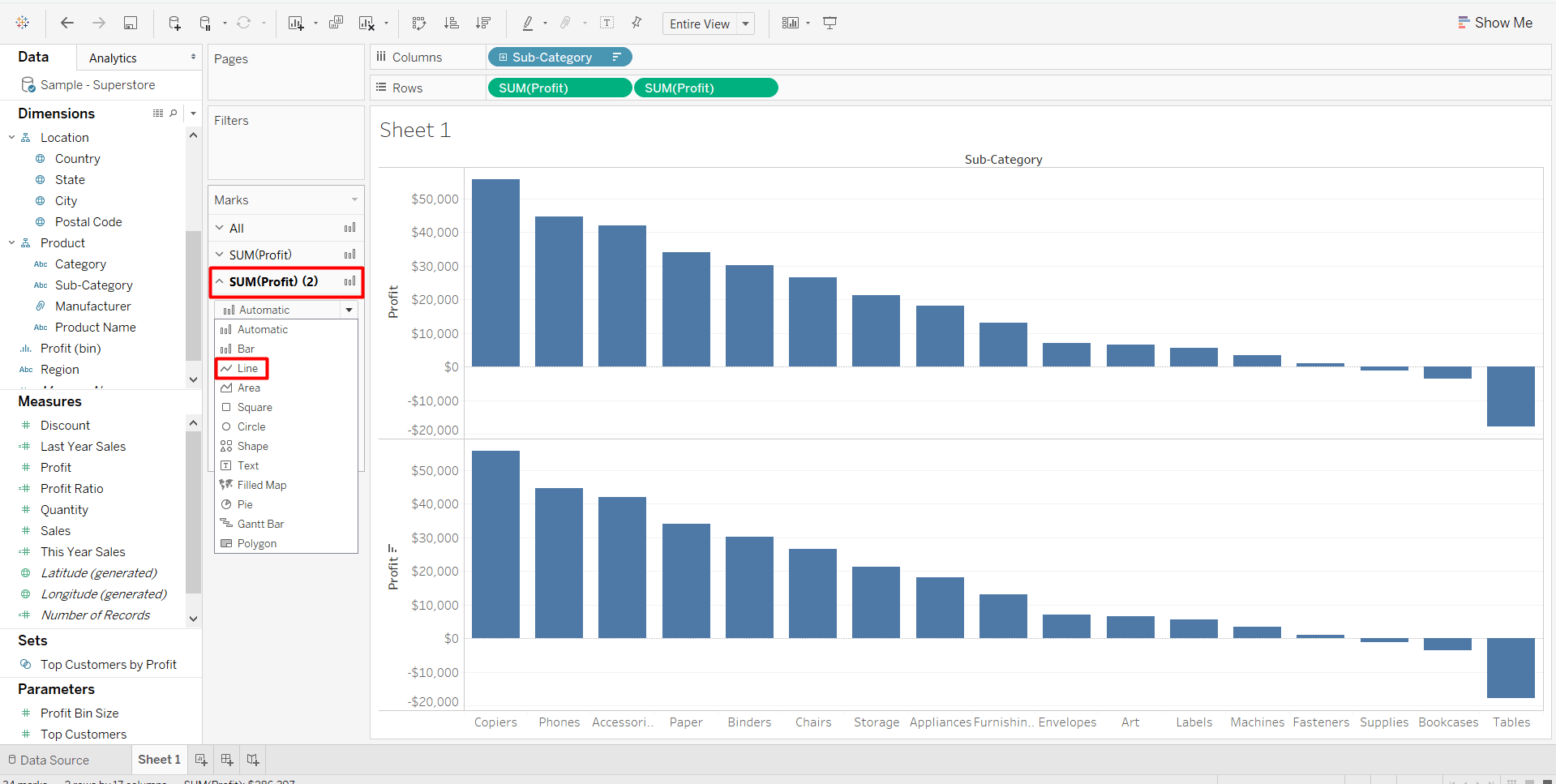7. Make a dual axis chart by selection the “Dual Axis” option from a 2nd copy of ‘Sum(Profit)” measure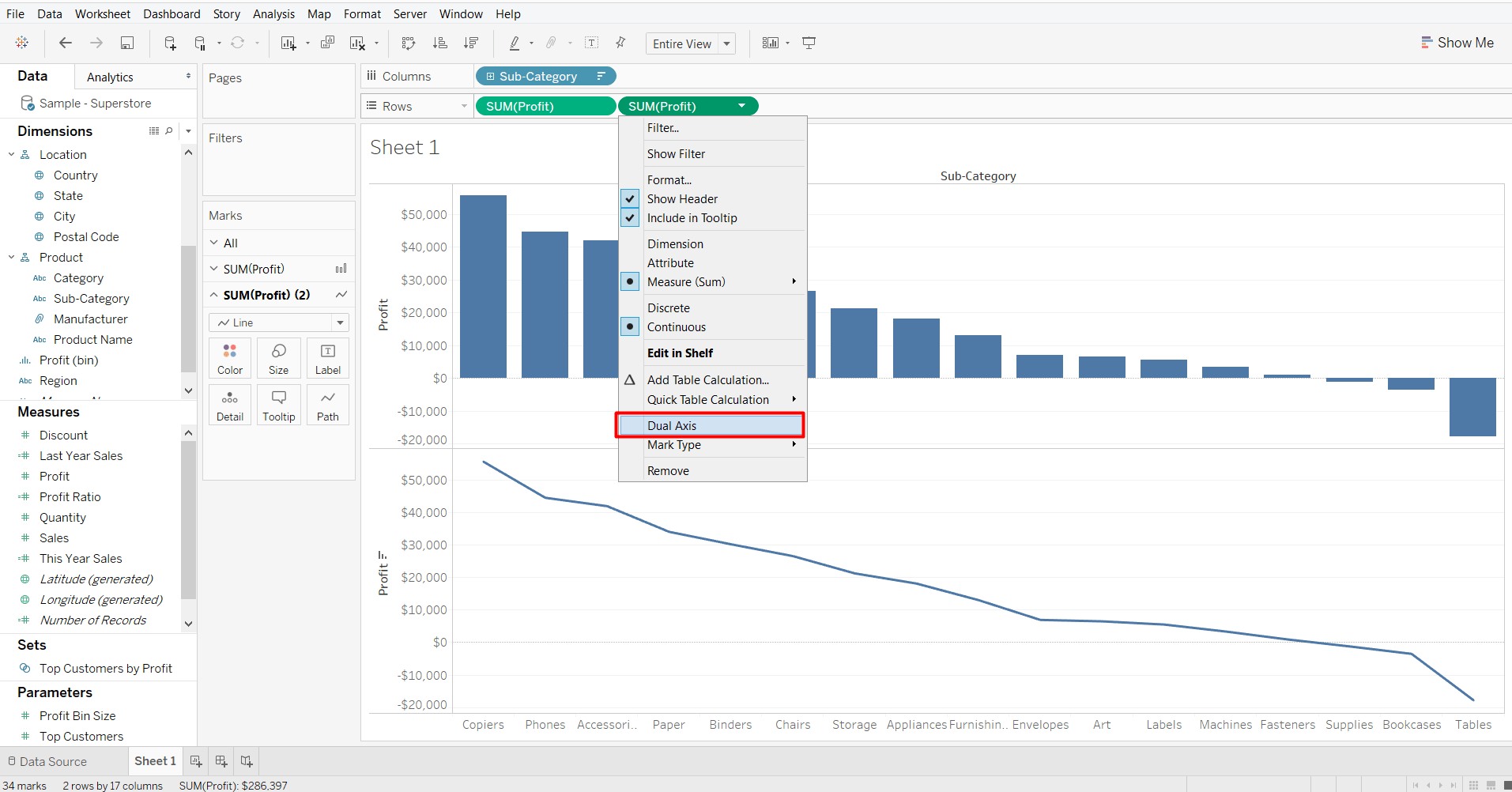8. Perform the table calculation on the 2nd copy of “Profit” field:
1. Add a “Running Total“ as Quick Table Calculation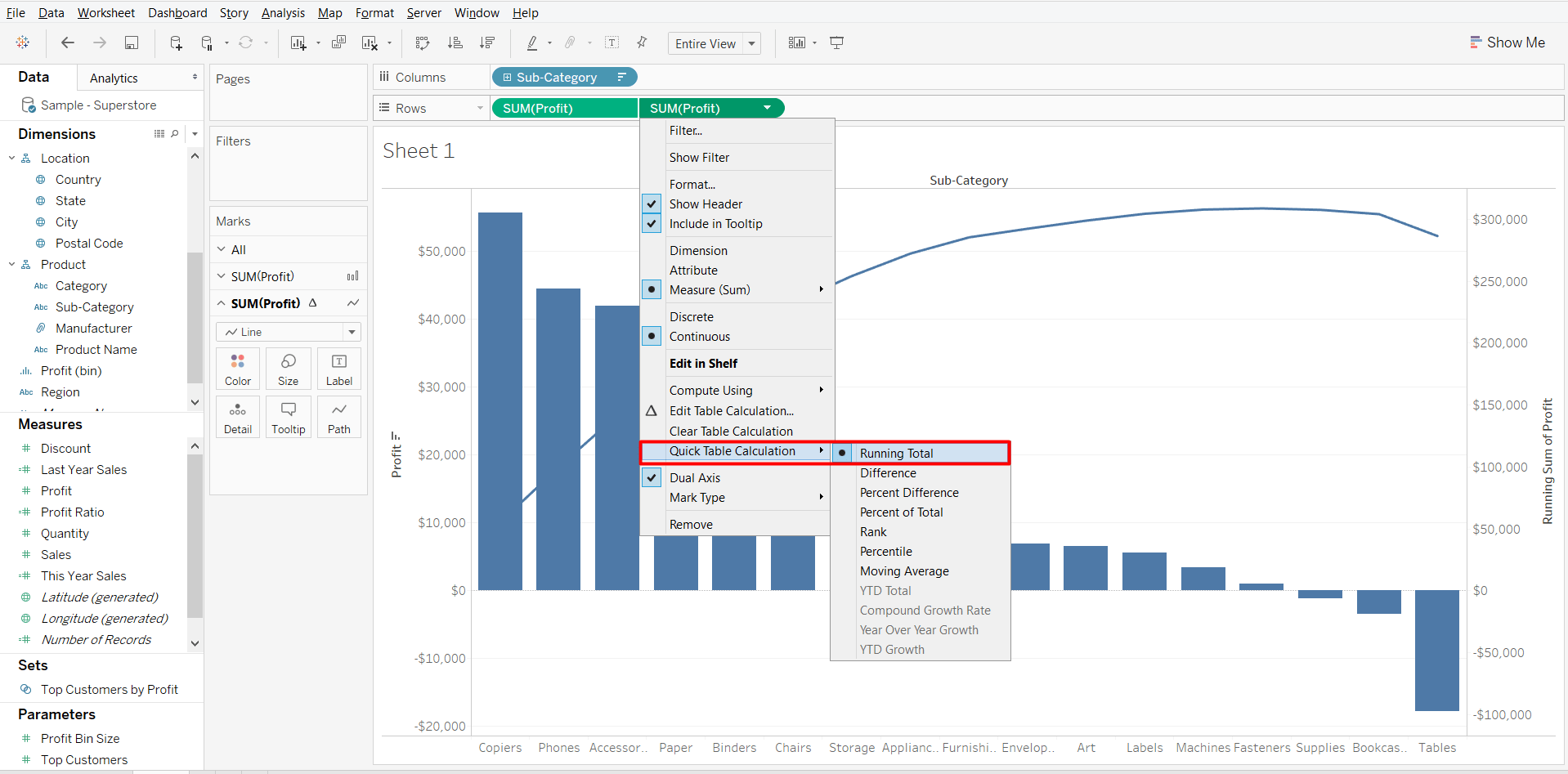2. Now, select “Edit table calculation” option3. Select “Add secondary calculation” and under “Secondary Calculation Type” select “Percent of Total” option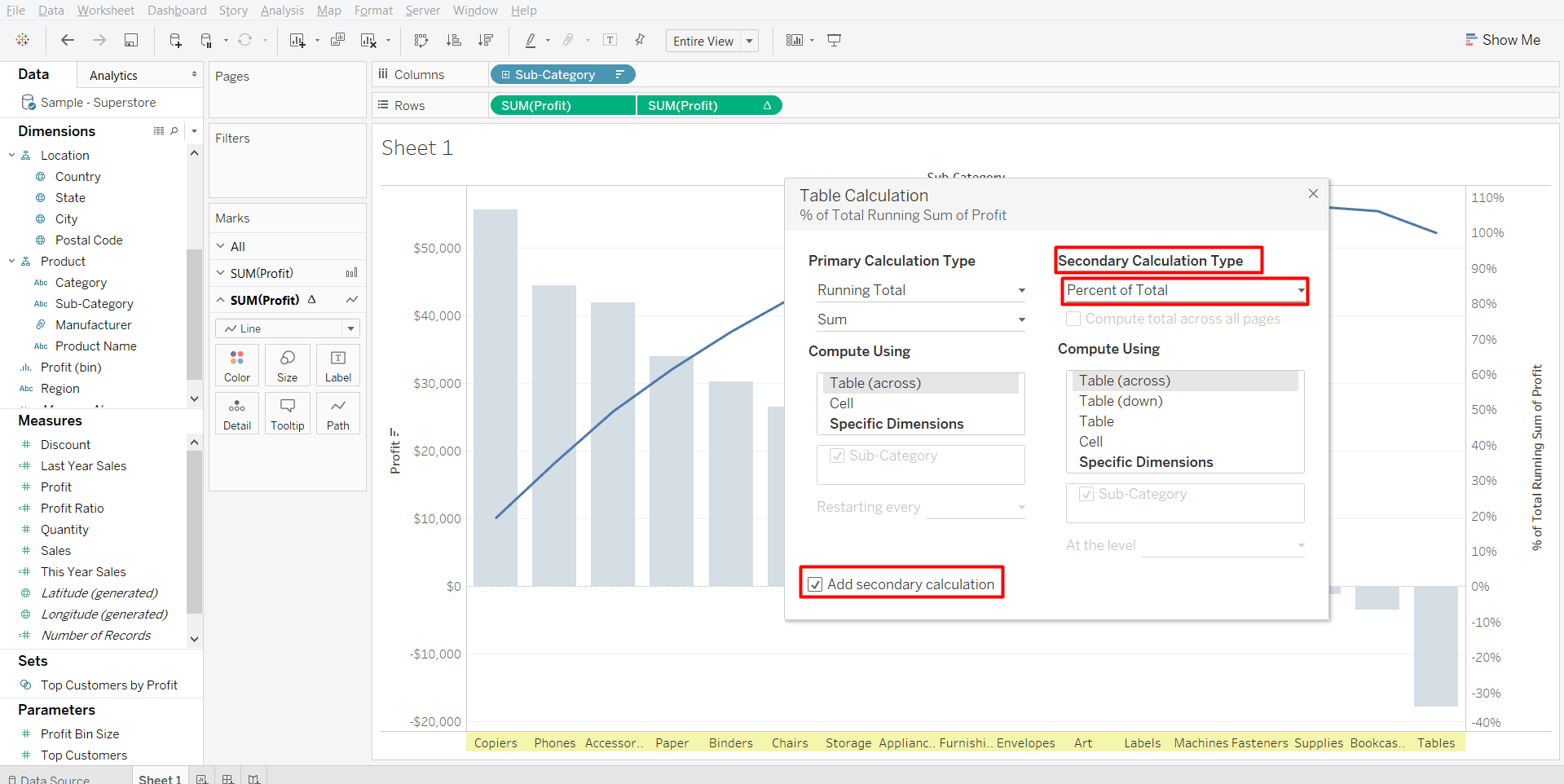9. Click on the “Color” marks card, to change the color of the line and the Pareto chart is completed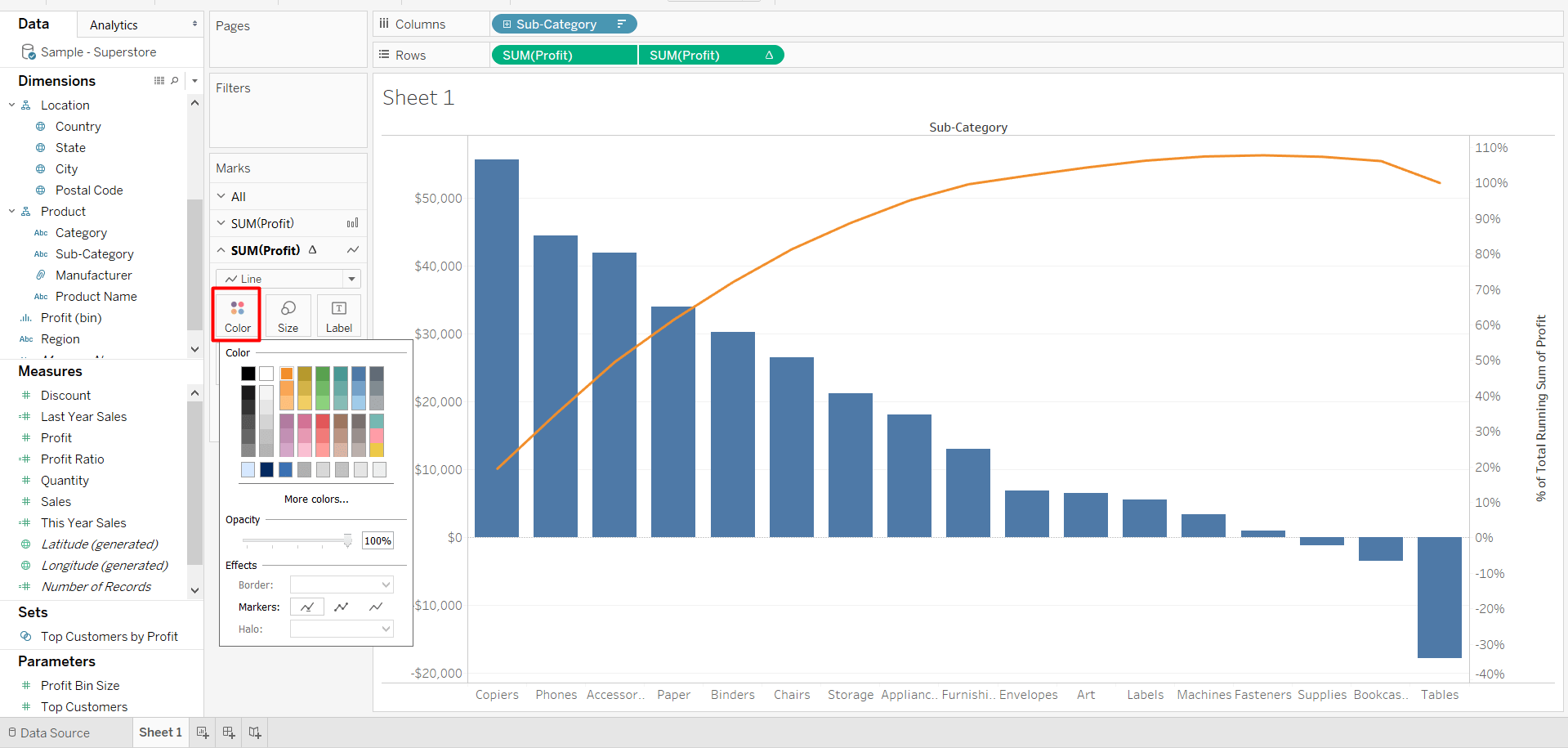• in
• |
• October 24, 2018#### Author1

Subscribe
Notify of